# MCQ in DC Circuits Part 5 | REE Board Exam

(Last Updated On: November 13, 2020)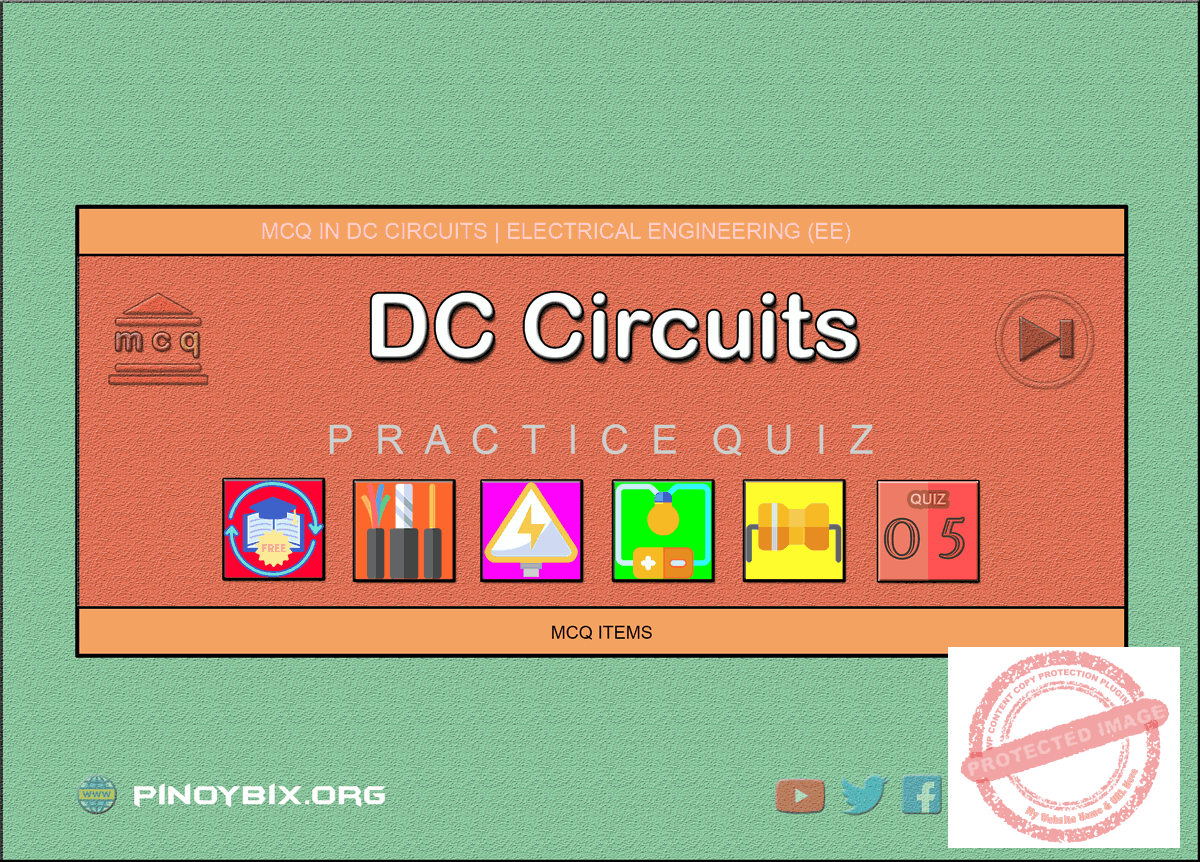This is the Multiple Choice Questions Part 5 of the Series in DC Circuits as one of the Electrical Engineering topic. In Preparation for the REE Board Exam make sure to expose yourself and familiarize in each and every questions compiled here taken from various sources including but not limited to past Board Exam Questions in Electrical Engineering field, Electrical Engineering Books, Journals and other Electrical Engineering References.

#### Continue Practice Exam Test Questions Part 5 of the Series

OHM’S LAW AND ELECTRIC CIRCUITS

Choose the letter of the best answer in each questions.

201.  The  hot  resistance  of  an  incandescent  lamp  is  10 ohms and the rated voltage is 50 V. Find the series resistance required to operate the lamp from an 80 V supply.

A.  8 Ω

B.  4 Ω

C.  6 Ω

D.  10 Ω

Solution:

202.  Ohm’s law is not applicable to

A.  copper

B.  silver

C.  silicon carbide

D.  aluminum

Solution:

203.  The practical unit of electrical energy is

A.  watt

B.  kilowatt

C.  kilowatt-hour

D.  megawatt

Solution:

204.  A 100 watt lamp working for 20 hours  will consume ____ units.

A.  200

B.  20

C.  2

D.  5

Solution:

205.  The  hot  resistance  of  an  incandescent  lamp  is about ____ its cold resistance.

A.  10 times

B.  2 times

C.  100 times

D.  50 times

Solution:

206.  A d.c. circuit usually has ______ as the load.

A.  resistance

B.  inductance

C.  capacitance

D.  both inductance and capacitance

Solution:

207.  The purpose of load in an electric circuit is to ______.

A.  increase the circuit current

B.  utilize electrical energy

C.  decrease the circuit current

D.  none of these

Solution:

208.  Electrical  appliances  are  not  connected  in  series because ______.

A.  series circuit is complicated

B.  appliances have different   current rating

C.  power loss is more

D.  none of these

Solution:

209.  Electrical  appliances  are  connected  in  parallel because it ______.

A.  is a simple circuit

B.  draws less current

C.  results in reduce in power loss

D.  makes the operation of appliances independent of each other

Solution:

210.  Inductance  and  capacitance  are  not  relevant  in  a d.c. circuit because ______.

A.  frequency of d.c. is zero

B.  it is a simple circuit

C.  they do not exist in a d.c. circuit

D.  none of these

Solution:

211.  The  hot  resistance  of  a  100  watt,  250  V incandescent lamp would be

A.  2.5 ohms

B.  625 ohms

C.  25 ohms

D.  none of these

Solution:

212.  The  voltage  drop  across  14.5  ohm  resistor  in  Fig. 2.1 is ______.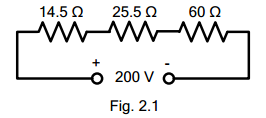A.  29 V

B.  14 V

C.  30.5 V

D.  18 V

Solution:

213.  The circuit shown in Fig. 2.1 is called a series circuit because ______.A.  it contains a few resistances

B.  it  carries  the  same  current  throughout  the circuit

C.  it is a simple circuit

D.  none of these

Solution:

214. Referring  to  Fig.  2.2,  the  total  circuit  resistance  will  be ______.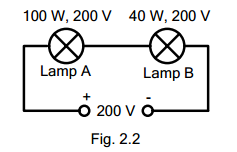A.  1000 ohms

B.  400 ohms

C.  1400 ohms

D.  135 ohms

Solution:

215.  In Fig. 2.2 ____.A.  the lamp A will be brighter than lamp B

B.  the lamp B will be brighter than lamp A

C.  the two lamps will be equally bright

D.  none of these

Solution:

216.  When  a  number  of  resistances  are  connected  in parallel, the total resistance is ______.

A.  less than the smaller resistance

B.  greater than the smaller resistance

C.  between the smaller and greatest resistance

D.  none of these

Solution:

217.  Two  resistances  of  6  ohms  and  3  ohms  are connected in parallel. The total resistance is ______.

A.  9 ohms

B.  18 ohms

C.  0.5 ohm

D.  2 ohms

Solution:

218.  The value of R that will give a total resistance of 1.5 ohms in Fig. 2.3 is _____.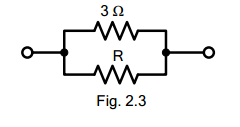A.  4 ohms

B.  6 ohms

C.  3 ohms

D.  9 ohms

Solution:

219.  The  current  in  2.5  ohm  resistor  in  Fig.  2.4  will  be ____.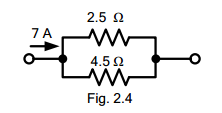A.  3 A

B.  4.5 A

C.  2.5 A

D.  2 A

Solution:

220.  The current in 4.5 ohms resistor in Fig. 2.4 will be ______.A.  3.5 A

B.  3 A

C.  2 A

D.  2.5 A

Solution:

221.  If  18  resistances,  each  of  value  36  ohms,  are connected  in  parallel,  then  the  total  resistance  is ______.

A.  2 ohms

B.  54 ohms

C.  36 ohms

D.  none of these

Solution:

222.  Two  incandescent  lamps  of  100  W,  200  V  are  in parallel  across  200  V  supply.  The  total  resistance will be ____.

A.  800 ohms

B.  200 ohms

C.  400 ohms

D.  600 ohms

Solution:

223.  The resistance across the terminals AB of the circuit shown in Fig. 2.5 is ______.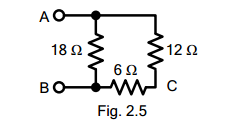A.  36 ohms

B.  18 ohms

C.  9 ohms

D.  15 ohms

Solution:

224.  If  a  d.c.  supply  of  180  V  is  connected  across terminals  AB  in  Fig.  2.5,  then  current  in  6  ohm resistor will be ____.A.  10 A

B.  5 A

C.  12 A

D.  6 A

Solution:

225.  The  resistance  across  terminals  AC  in  Fig.  2.5  is ______.A.  36 ohms

B.  9 ohms

C.  18 ohms

D.  8 ohms

Solution:

226.  The  resistance  across  terminals  AB  of  the  circuit shown in Fig. 2.6 is ______.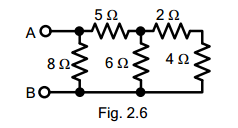A.  4 ohms

B.  18 ohms

C.  34 ohms

D.  8 ohms

Solution:

227.  If a battery of 24 V is applied across terminals AB of the circuit shown in Fig. 2.6, then current in 2 ohm resistor will be ____.A.  3 A

B.  6 A

C.  2.5 A

D.  1.5 A

Solution:

228.  If a battery of 24 V is applied across terminals AB in Fig. 2.6, then power loss in 5 ohms resistor will be ______.A.  180 W

B.  45 W

C.  90 W

D.  24 W

Solution:

229.  The  total  conductance  of  the  circuit  shown  in  Fig. 2.7 is _____.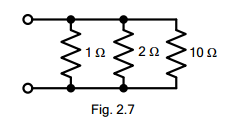A.  13 mhos

B.  1.6 mhos

C.  6 mhos

D.  2.5 mhos

Solution:

230.  If  10  ohms  resistance  is  removed  in  Fig.  2.7,  then total conductance of the circuit will be _____.A.  3 mhos

B.  6 mhos

C.  2 mhos

D.  1.5 mhos

Solution:

231.  The voltage across the parallel circuit shown in Fig. 2.8 is _____.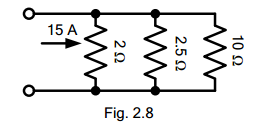A.  15 V

B.  10 V

C.  30 V

D.  12. 5 V

Solution:

232.  The current in 10 ohms resistor in Fig. 2.8 is ______.A.  3 A

B.  2.5 A

C.  1.5 A

D.  3.5 A

Solution:

233.  The  total  resistance  between  terminals  1  and  2  of the circuit shown in Fig. 2.9 is _____.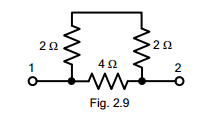A.  12 ohms

B.  2.67 ohms

C.  2 ohms

D.  64 ohms

Solution:

234.  If a battery of 12 V is applied across terminals 1 and 2 of Fig. 1.9, then current through 4 ohms resistor will be _____.A.  1.5 A

B.  3 A

C.  2 A

D.  2.5 A

Solution:

235.  The  resistance  between  terminals  1  and  2  of  Fig. 1.10 is ______.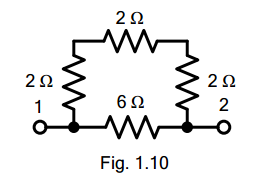A.  12 ohms

B.  8 ohms

C.  16 ohms

D.  3 ohms

Solution:

236.  The  resistance  between  terminals  1  and  2  in  Fig. 1.11 is ______.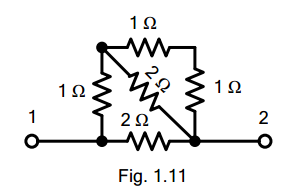A.  2 ohms

B.  1.5 ohms

C.  1 ohm

D.  4 ohms

Solution:

237.  If  a battery of 6 V is applied across terminals 1 and 2 in Fig. 1.11, then current in the horizontal 2 ohm resistor will be _____.A.  1 A

B.  2 A

C.  3 A

D.  0.5 A

Solution:

238.  The resistance across terminals 1 and 2 in Fig. 1.12 is ____.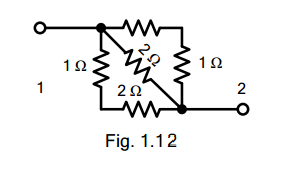A.  6 ohms

B.  12 ohms

C.  18 ohms

D.  24 ohms

Solution:

239.  Two  equal  resistances  are  connected  in  series across a certain supply. If the resistances are now connected  in  parallel  across  the  same  supply,  the power  produced  will  be  ____  that  of  series connection.

A.  two times

B.  four times

C.  one-half

D.  one-fourth

Solution:

240.  Referring  to  Fig.  1.13,  the  resistance  across terminals BE is _____.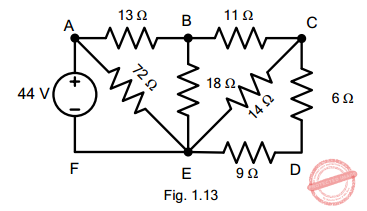A.  9 ohms

B.  18 ohms

C.  10 ohms

D.  none of these

Solution:

241.  Referring  to  Fig.  1.13,  the  resistance  across terminals AF is ______.A.  20.5 ohms

B.  18 ohms

C.  11 ohms

D.  none of these

Solution:

242.  Referring  to  Fig.  1.13,  the  current  in  18  ohms resistor will be _____.A.  2 A

B.  1.5 A

C.  1 A

D.  none of these

Solution:

243.  Referring  to  Fig.  1.13,  the  power  loss  in  11  ohms will be _____.A.  11 W

B.  24 W

C.  16 W

D.  none of these

Solution:

244.  If in Fig. 1.14, switches S1  and S2  are closed, then total circuit resistance is _____.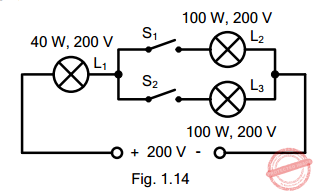A.  400 ohms

B.  1200 ohms

C.  1000 ohms

D.  2400 ohms

Solution:

245.  If switch S1  is open and switch S is closed in Fig. 1.14, then circuit resistance will be _____.A.  1200 ohms

B.  1000 ohms

C.  1400 ohms

D.  2400 ohms

Solution:

246.  If in Fig. 1.14, both switches S1  and S2  are closed, then _____.A.  L1 will be brighter than L2 or L3

B.  L1 will be dimmer than L2 or L3

C.  L1 will be as bright as L2 or L3

D.  none of these

Solution:

247.  If  in  Fig.  1.14  switches  S1  and  S2  are  open,  then lamp L1 will give output ______.A.  less than 40 W

B.  more than 40 W

C.  equal to 40 W

D.  none of these

Solution:

248.  If in Fig. 1.14 switches S1 and S2 are closed and the supply voltage is increased to 400 V, then _____.A.  lamp L1 will burn out

B.  lamp L2 will burn out

C.  both lamps L2 and L3 will burn out

D.  all the lamps will be safe

Solution:

249.  If  in  Fig.  1.1,  resistor  R2  becomes  open-circuited, the reading of the voltmeter will become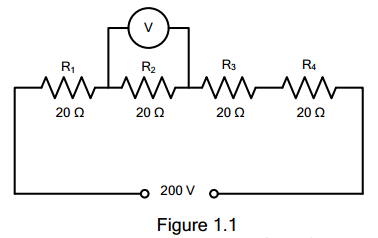A.  zero

B.  150 V

C.  50 V

D.  200 V

Solution:

250.  Whatever the battery voltage in Fig. 1.2, it is certain that  smallest  current  will  flow  in  the  resistance  of _____ ohm.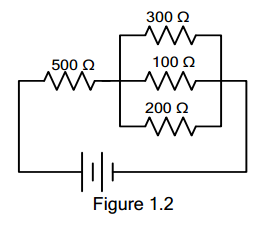A.  300

B.  500

C.  200

D.  100

Solution:

#### Questions and Answers in DC Circuits

Following is the list of practice exam test questions in this brand new series:

MCQ in DC Circuits
PART 1: MCQ from Number 1 – 50                Answer key: included
PART 2: MCQ from Number 51 – 100            Answer key: included
PART 3: MCQ from Number 101 – 150           Answer key: included
PART 4: MCQ from Number 151 – 200           Answer key: included
PART 5: MCQ from Number 201 – 250           Answer key: included
PART 6: MCQ from Number 251 – 300           Answer key: included
PART 7: MCQ from Number 301 – 350           Answer key: included
PART 8: MCQ from Number 351 – 400            Answer key: included
PART 9: MCQ from Number 401 – 450            Answer key: included
PART 10: MCQ from Number 451 – 500          Answer key: included

P inoyBIX educates thousands of reviewers and students a day in preparation for their board examinations. Also provides professionals with materials for their lectures and practice exams. Help me go forward with the same spirit.

“Will you subscribe today via YOUTUBE?”

Subscribe

PinoyBIX Engineering. © 2014-2020 All Rights Reserved | How to Donate? |